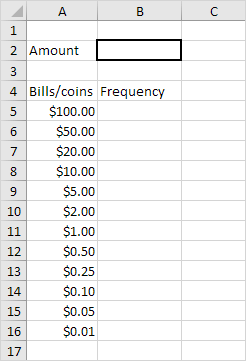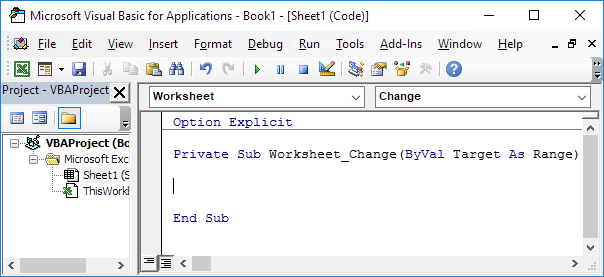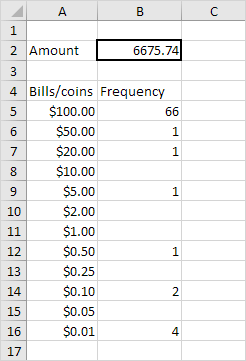# Bills and Coins

Below we will look at a program in Excel VBA that splits an amount of money into bills and coins.

Situation:Create a Worksheet Change Event. Code added to the Worksheet Change Event will be executed by Excel VBA when you change a cell on a worksheet.

1. Open the Visual Basic Editor.

2. Double click on Sheet1 (Sheet1) in the Project Explorer.

3. Choose Worksheet from the left drop-down list. Choose Change from the right drop-down list.Add the following code lines to the Worksheet Change Event:

4. Declare a variable called amount of type Double and a variable i of type Integer.

Dim amount As Double, i As Integer

5. The Worksheet Change Event listens to all changes on Sheet1. We only want Excel VBA to do something if something changes in cell B2. To achieve this, add the following code line:

6. We initialize the variable amount with the value of cell B2.

amount = Range("B2").Value

7. We empty the range with the frequencies.

Range("B5:B16").Value = ""

8. Now it's time to split the entered amount of money. We start a For Next loop.

For i = 5 To 16

9. We will make use of the Do While Loop structure. Code placed between these words will be repeated as long as the part after Do While is true. We want Excel VBA to repeat the code lines at step 10 as long as amount is larger or equal to Cells(i,1).value.

Do While amount >= Cells(i, 1).Value

Loop

10. Add the following code lines to the Do While Loop.

Cells(i, 2).Value = Cells(i, 2).Value + 1
amount = amount - Cells(i, 1).Value

Explanation: as long as amount is larger or equal to Cells(i,1).value, the amount contains bills/coins of this value. As a result, Excel VBA increments the frequency of this bill/coin (first line) and subtracts the value of the bill/coin from amount (second line). This process will be repeated until amount becomes smaller than Cells(i,1).value. Next, Excel VBA increments i and goes to the next bill/coin to see how many times this bill/coin fits in the amount left. This way the amount of money will be split into bills and coins until there is no money left to split anymore.

11. Close the For Next loop and don't forget to close the if statement (both outside the Do While Loop).

Next i
End if

12. Test the program.

Result:Note: of course the entered amount does not necessarily contains every bill/coin. If amount does not contain a certain bill/coin, the part after Do While never becomes true for this bill/coin and Excel VBA goes directly to the next bill/coin.

Go to Next Chapter: Array